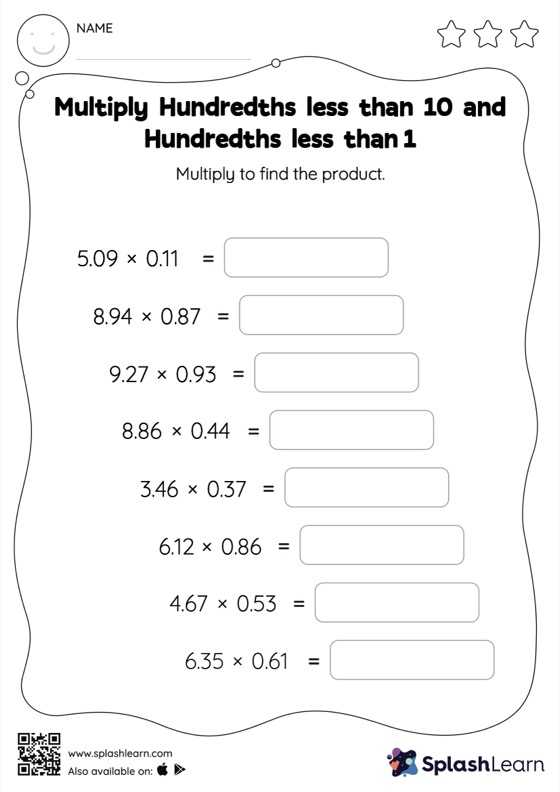# Multiply Hundredths less than 10 and Hundredths less than 1: Horizontal Multiplication Worksheet

Home > Multiply Hundredths less than 10 and Hundredths less than 1: Horizontal MultiplicationCan your young mathematician multiply hundredths less than 10 and hundredths less than 1? That's exactly what they do here. Students first multiply as though there is no decimal when multiplying two decimals. They then add the same number of digits after the decimal point in the product as there are decimal digits in each number. This multiply hundredths less than 10 and hundredths less than 1 worksheet gives students a thorough practice of this concept.